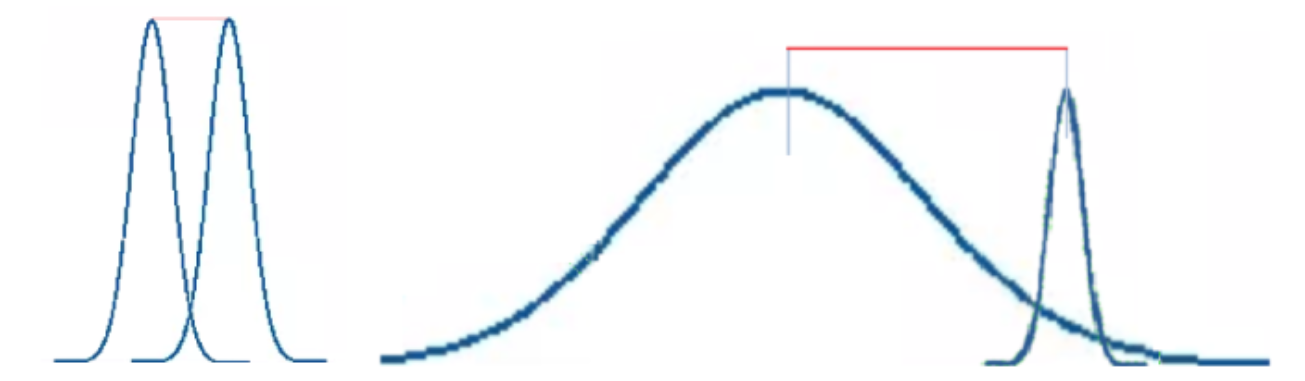# Definition

The Student's t-test is used to compare two means from two independent groups. The conditions of application for this test are : the data have to follow a normal distribution (usually the number of participants per group has to be > 30) and the groups have to get similar variances.

# In practice

The Student's t-test is commonly used to compare the mean of a variable between two groups. For example, "pain score" mean difference between the groups "treated" versus "untreated". The groups need to be similar in variance, i.e. the two groups should have a similar repartition of the patients around the mean of the group. If this is not the case, the comparison risks to be unreliable.It is easiest to compare the two curves on the left (no difference in variances) than the two curves on the rigth (difference in variances).

# How to use it on EasyMedStat ?

1. Go to Statistics > Test variables
2. Select a Numeric variable
3. Select a Yes-No variable to create groups of patients
4. Click on "Which tests can I do ?"
5. Click on "Compare values of ..."